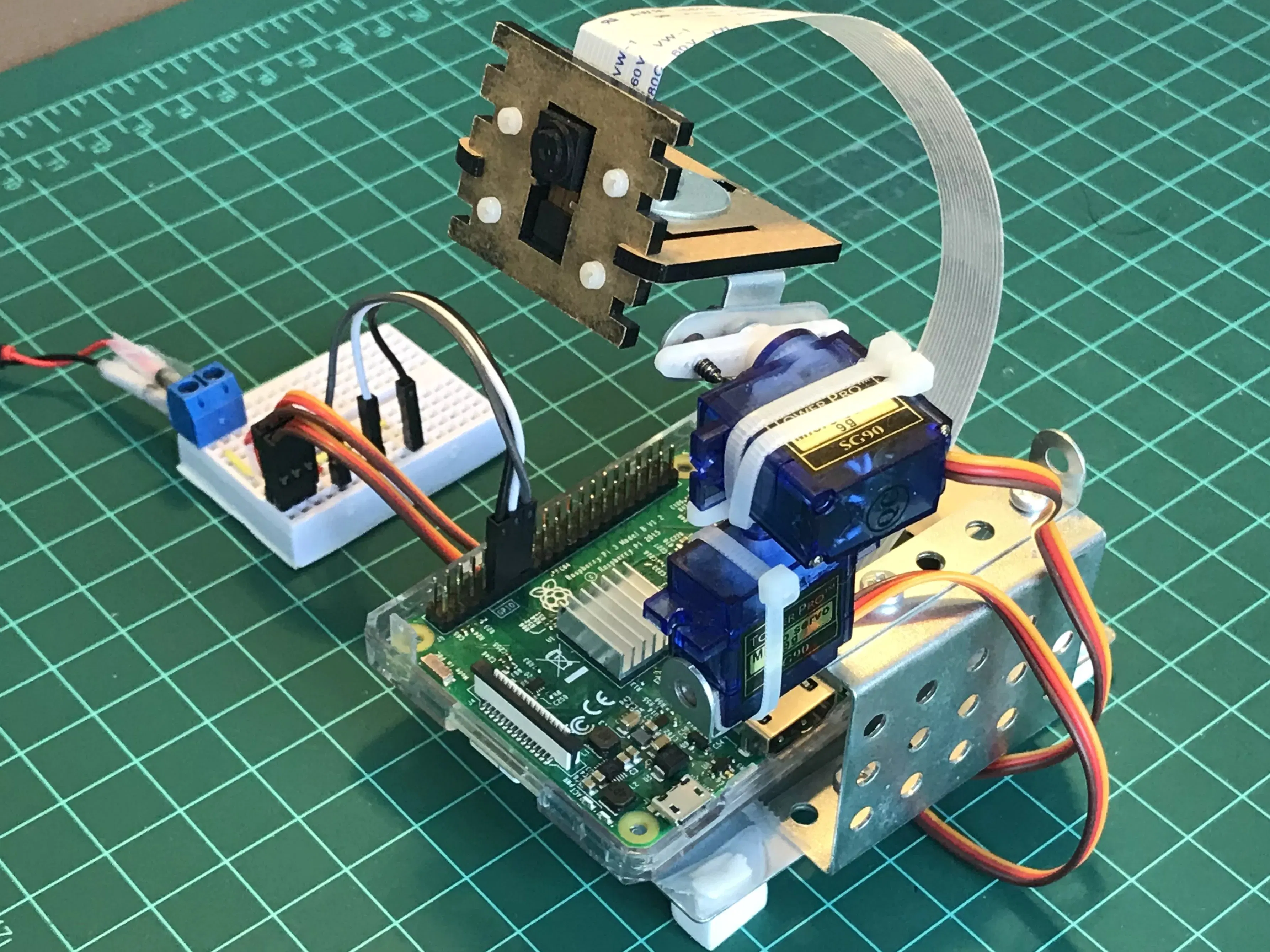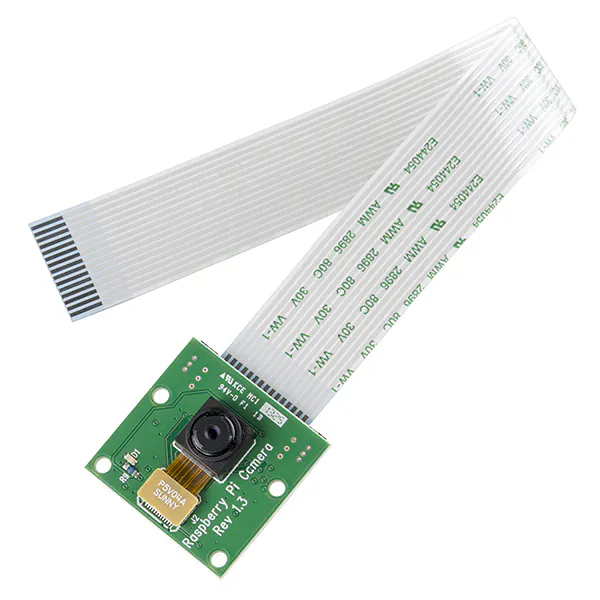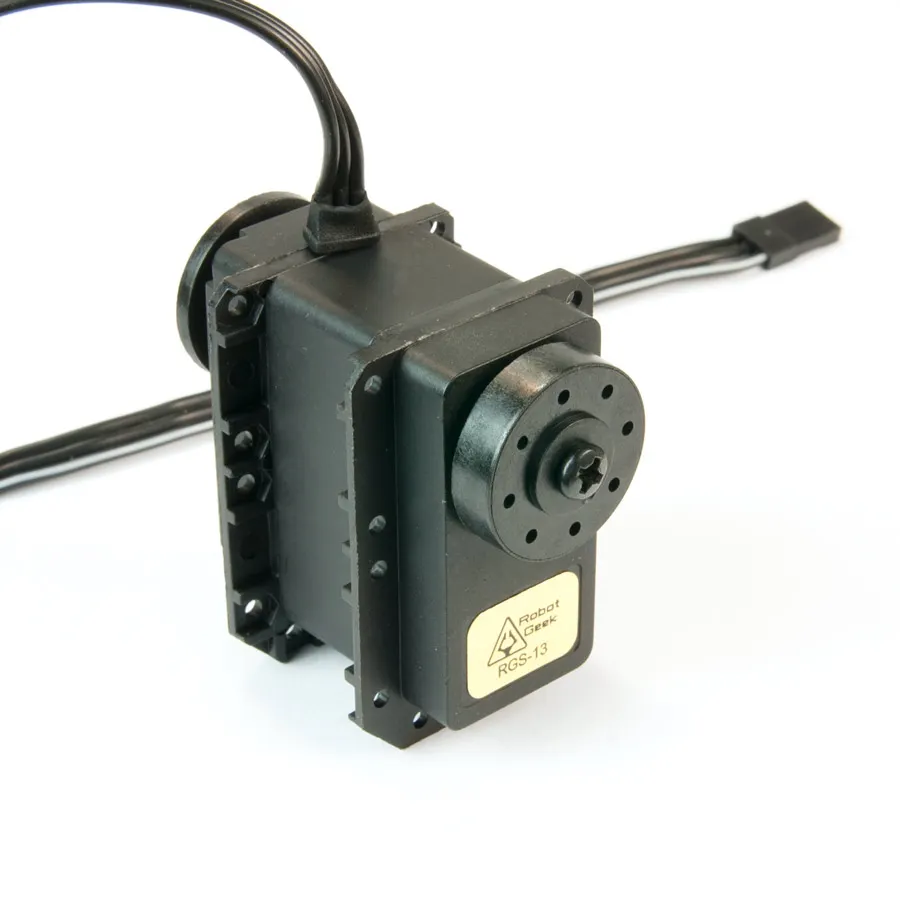# Pan-Tilt Multi Servo Control

Multiple servos control, using Python and a PAN/TILT mechanism construction to PiCam positioning.

BeginnerFull instructions provided8 hours19,174## Things used in this project

### Hardware componentsRaspberry Pi 3 Model B
×1Raspberry Pi Camera Module
×1RobotGeek 180 Degree Robot Servo
×2

### Software apps and online servicesRaspberry Pi Raspbian

## Code

### Code snippet #13

Plain text
```from time import sleep
import RPi.GPIO as GPIO
GPIO.setmode(GPIO.BCM)
GPIO.setwarnings(False)

def setServoAngle(servo, angle):
pwm = GPIO.PWM(servo, 50)
pwm.start(8)
dutyCycle = angle / 18. + 3.
pwm.ChangeDutyCycle(dutyCycle)
sleep(0.3)
pwm.stop()

if __name__ == '__main__':
import sys
servo = int(sys.argv)
GPIO.setup(servo, GPIO.OUT)
setServoAngle(servo, int(sys.argv))
GPIO.cleanup()
```

### Code snippet #15

Plain text
```from time import sleep
import RPi.GPIO as GPIO
GPIO.setmode(GPIO.BCM)
GPIO.setwarnings(False)

pan = 27
tilt = 17

GPIO.setup(tilt, GPIO.OUT) # white => TILT
GPIO.setup(pan, GPIO.OUT) # gray ==> PAN

def setServoAngle(servo, angle):
assert angle >=30 and angle <= 150
pwm = GPIO.PWM(servo, 50)
pwm.start(8)
dutyCycle = angle / 18. + 3.
pwm.ChangeDutyCycle(dutyCycle)
sleep(0.3)
pwm.stop()

if __name__ == '__main__':
import sys
if len(sys.argv) == 1:
setServoAngle(pan, 90)
setServoAngle(tilt, 90)
else:
setServoAngle(pan, int(sys.argv)) # 30 ==> 90 (middle point) ==> 150
setServoAngle(tilt, int(sys.argv)) # 30 ==> 90 (middle point) ==> 150
GPIO.cleanup()
```

### Code snippet #17

Plain text
```from time import sleep
import RPi.GPIO as GPIO
GPIO.setmode(GPIO.BCM)
GPIO.setwarnings(False)

pan = 27
tilt = 17

GPIO.setup(tilt, GPIO.OUT) # white => TILT
GPIO.setup(pan, GPIO.OUT) # gray ==> PAN

def setServoAngle(servo, angle):
assert angle >=30 and angle <= 150
pwm = GPIO.PWM(servo, 50)
pwm.start(8)
dutyCycle = angle / 18. + 3.
pwm.ChangeDutyCycle(dutyCycle)
sleep(0.3)
pwm.stop()

if __name__ == '__main__':
for i in range (30, 160, 15):
setServoAngle(pan, i)
setServoAngle(tilt, i)

for i in range (150, 30, -15):
setServoAngle(pan, i)
setServoAngle(tilt, i)

setServoAngle(pan, 100)
setServoAngle(tilt, 90)
GPIO.cleanup()
```

### Github file

https://github.com/Mjrovai/RPi-Pan-Tilt-Servo-Control/blob/master/PanTiltControl/angleServoCtrl.py

## Credits

### MJRoBot (Marcelo Rovai)

39 projects • 729 followers
Professor, Engineer, MBA, Master in Data Science. Writes about Electronics with a focus on Physical Computing, IoT, ML, TinyML and Robotics.